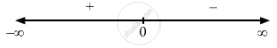Share

F(X) = 1 X 2 + 2 . - CBSE (Arts) Class 12 - Mathematics

Question

f(x) = $\frac{1}{x^2 + 2}$ .

Solution

$\text { Given }: \hspace{0.167em} f\left( x \right) = \frac{1}{x^2 + 2}$

$\Rightarrow f'\left( x \right) = \frac{- 2x}{\left( x^2 + 2 \right)^2}$

$\text { For the local maxima or minima, we must have }$

$f'\left( x \right) = 0$

$\Rightarrow \frac{- 2x}{\left( x^2 + 2 \right)^2} = 0$

$\Rightarrow x = 0$Now, for values close to x = 0 and to the left of 0,

$f'\left( x \right) > 0$ .
Also, for values close to x = 0 and to the right of 0, $f'\left( x \right) < 0$ .
Therefore, by first derivative test, x = 0 is a point of local maxima and the local maximum value of
$f\left( x \right) \text { is }\frac{1}{2} .$
Is there an error in this question or solution?

Video TutorialsVIEW ALL 

Solution F(X) = 1 X 2 + 2 . Concept: Graph of Maxima and Minima.
S